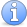## Combinatorics front page

### Quick descriptionIncomplete This article is incomplete. We need a description of algebraic combinatorics and we need to create the two missing front pages. It might be good to have a paragraph or so on what combinatorics is.

Additive combinatorics Quick description. ( Additive combinatorics is a difficult area to define. The basic object of study could be said to be finite subsets of Abelian groups, but the subject is characterized more by its techniques than by its subject matter. These techniques are a blend of ideas from combinatorics, harmonic analysis, ergodic theory, and analytic number theory. )

Algebraic combinatorics

Enumerative combinatorics Quick description. ( Enumerative combinatorics is what many people understand by "combinatorics": it concerns exact counting of combinatorial structures of various kinds. The meaning of "exact counting" varies from problem to problem. The ideal is a simple formula for the number of structures, but other possibilities are recurrence relations for the number of structures associated with a parameter, generating functions for this number, or efficient algorithms for computing the number. Sometimes two very different combinatorial structures give rise to the same formula. Another goal of enumerative combinatorics is to explain such apparent coincidences by finding natural bijections between the two types of structures. )

Extremal combinatorics Quick description. ( The branch of combinatorics where one would like to maximize or minimize some parameter associated with a combinatorial structure, subject to certain constraints.)

Probabilistic combinatorics Quick description. ( Probabilistic combinatorics refers to three activities: the use of randomized constructions to prove the existence of structures with given properties, the use of random methods to prove deterministic theorems, and the study of random structures for their own sake.)

Structural graph theory Quick description. ( This area includes famous results such as the four-colour theorem, the Robertson-Seymour theorem and the strong perfect graph theorem.)